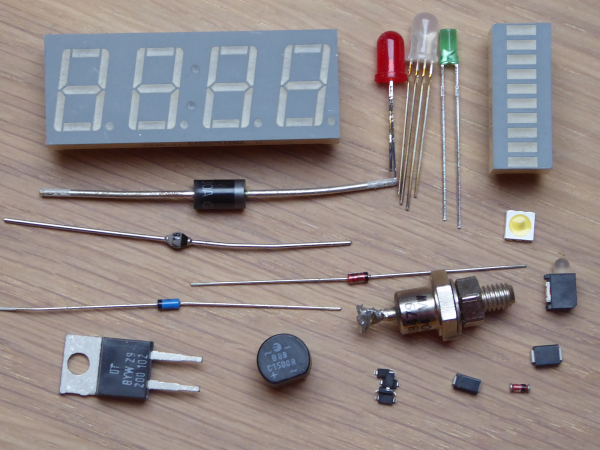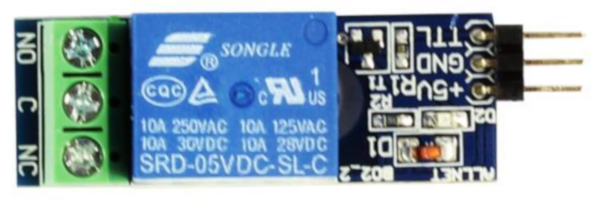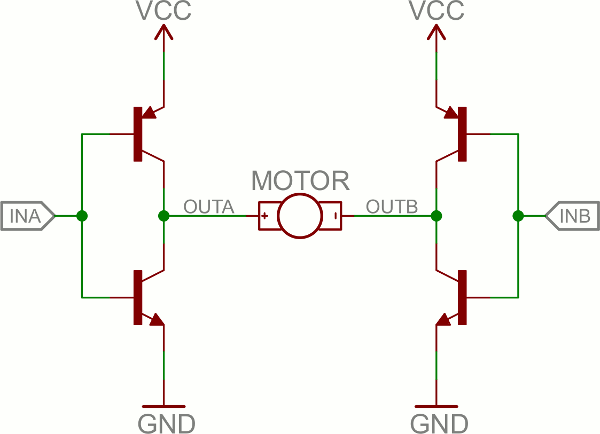# Tutorials: Electronics fundamentals (ELEFU)

## Diode D and Transistor T

last updated: 2021-02-01

### Introduction

Song of this chapter: Aerosmith > Aerosmith > One way street

After the discovery of rectifying properties and the Edison effect, and the development of diodes (both vacuum-tube and semiconductor) and triodes (vacuum-tube), it was possible to get a DC voltage and current from an AC source, and to build radio receiver detectors and amplifiers like the crystal detector used in early 20th century radio receivers.

In the midst of the 20 century semiconductor diodes and transistors based on germanium and silicon replaced the vacuum tubes, consuming less energy and volume. Now it was possible to produce portable electronic devices. Today most diodes and transistors are based on silicon, but germanium and gallium arsenide are also used.

Beginning in the 1970s, more and more transistors were connected on a single chip (integrated circuits IC or chip). With this began the transistor count in IC's.

### Diode (wiki)

Song of this chapter: Aerosmith > Aerosmith > One way street

Diodes are non-linear components and they are always polarized!

They let current flow in only one direction! For this they have to be forward-biased, meaning there is a positive potential on one terminal, called anode and a negative potential on the other terminal called cathode. The potential difference must be greater than approximately 0.7 V for silicon diodes (0.3 V for germanium diodes and 0.2 V for Schottky diodes).#### Water analogy

In the water analogy we have a one-way valve. A spring shows that we need a certain pressure (voltage) to open the door if the water flow is in forward direction.#### Diodes symbols and models

Symbol of a diode, a light emitting diode (LED) and a Zener diode (Z-diode):The symbol clearly shows the forward direction with an arrow. The cathode is shown with a line. In cylindrical housings we have a ring showing the cathode, on surface mount diodes (SMD) it's a line.

Standard LED's have often a longer leg indicating the anode pin or a flat edge on the LED’s outer casing showing the cathode pin. An easy way is also to use the diode function on a multi-meter to test for polarity (see later).#### Diode forward-biased and reverse-biased

At a greater voltage than 0.7 V the resistance of a silicon diode will get very low. With no limit for the current, the diode will eventually melt from the heat generated. So we have to include some other limiting component (e.g. resistor) to the circuit.#### p-n junction (wiki)

Silicon is basically found in sand and it's the the eighth most common element in the universe by mass. In it's pure form it's a hard and brittle crystalline solid. At -273 °C it's an insulator. But at 20 °C (+293K) we get a semiconductor because bonds in the crystal are breaking and we get free electrons.

By adding impurities to the pure silicon, different properties can be realized. Doping silicon with per example phosphorus introduces extra electrons. We get an n-type semiconductor that has extra electrons that can wander around the crystalline structure of the silicon. Doping silicon with per example boron creates a p-type semiconductor with missing electrons (holes) to complete the crystalline bonds.

Joining n-type silicon to p-type silicon creates a p-n junction. This p-n junction acts as a diode, or if the dimensions and doping are right as a photo cell.The junction between the types creates a barrier to the electrons and holes (because they are recombining we get an insulating zone), the depletion zone. In forward-bias direction a positive voltage on the anode (p-type), pulls the electrons to the junction and a negative voltage on the cathode (n-type) the holes. The depletion zone gets smaller. When the voltage difference is big enough the depletion zone disappears. The current can flow freely across the junction.

Reverse-biasing the p-n junction only widens the depletion zone. If the reverse-bias voltage difference is too high, the junction will heat up and we get an electrical_breakdown. This can destroy the p-n-junction.

#### Circuit example: AND and OR gates with diodes

In the last decades electronics moved dramatically from analogue to digital. Two elementary digital circuits are the `OR` gate and the `AND` gate. We will use the positive logic, so the two digital states `true` and `false` will be represented with `HIGH` (`1`, `+5V` or `+3.3V`) and `LOW` (`0`, `0V`). Two inputs for the gate will give 2² = 4 possibilities or cases (3 inputs would give 2³ = 8 cases).

##### Logic OR

Logic `OR` is only `false` if none of the conditions are fulfilled. If I drink water `OR` lemonade I won't be thirsty any more. It's not an exclusive OR because I can also drink both drinks, even mixed :). This gives us the following truth table:

case B A Z        case B A Z
1 false false false        1 0 0 0
2 false true true        2 0 1 1
3 true false true        3 1 0 1
4 true true true        4 1 1 1
##### Logic AND

Logic `AND` is only `true` if both conditions are fulfilled. If I have money `AND` my girlfriend has time we will go to the cinema.

case B A Z        case B A Z
1 false false false        1 0 0 0
2 false true false        2 0 1 0
3 true false false        3 1 0 0
4 true true true        4 1 1 1
###### "Just do it" D1:
• We will use diodes to build an `OR` gate and an `AND` gate. The output will be visualized with a light emitting diode. Build the first circuit and document all 4 states by drawing the 4 circuits with their corresponding measured potentials and currents. Explain how the circuit is working.• Build the second circuit and document all 4 states by drawing the 4 circuits with their corresponding measured potentials and currents. Explain how the circuit is working.

### Diode characteristics

Do you remember the last exercise in the second chapter (Ohm's law)? We measured the I-U-diagram of three different resistors and of our diode 1N4148.

The resistor has a linear characteristic! You can see in the I=f(U) diagram that when the voltage is doubled the corresponding current doubles. This is true for both directions, as the resistor is not polarized. Here is the straight from a resistor (1kΩ) in a complete diagram. In fact we combine two diagrams, one in forward-bias and one reverse-bias.Let's look at the diagram of a diode 1N4148. The 1N4148 is a standard silicon small signal fast switching diode. It's very often used in circuits, because of it's switching capabilities up to about 100 MHz. Because of it's popularity there are more than one manufacturer and also slightly different data-sheets.

Both diagrams are non-linear. Watch for the axis, they are not the same!! The diode is used in forward direction. Reverse biased it will break down (it will be destroyed) at 75 - 100 V.Between the two knees of the curve (UF and UBR) the current is practically zero. If the voltage gets higher than UF = 0.7 V the diode is getting conductive and the current is very soon reaching it's maximum (about 200 mA). That's why we need a another component in series (resistor) to limit the current and prevent the diode from being destroyed.

The other knee of the curve shows at witch voltage the diode breaks down (is also destroyed except for Zener diodes (see later)). This happens at UBR = 75 V-100 V.

###### "Just do it" D2:
• Look at the absolute maximum ratings in the data sheet of the diode 1N4148 and compare with our diagram. Also look at the diagrams in the data sheet. What are your findings?
Tip: There are more than one manufacturer and so also different data sheets. Search for the best data sheet suited.
• How does the temperature affects the curves of a diode?
• The forward voltage of a silicon diode lies between 0.5 V and 1 V. It can be measured approximately with a multi-meter. Measure the resistance of a diode 1N4148 in forward- and reverse-bias (select the range manually!) and measure the forward voltage with the multi-meter.As our characteristic curve is non-linear, it is difficult to calculate the currents and voltages in a circuit. Also the real curve may differ from the curve in our data sheet. A simple possibility is to measure the curve, draw a diagram and read the working point of the circuit from that diagram.

Here is an example for a diode 1N4148 in series with a resistor. The curve from the resistor is mirrored to get the working point of the circuit. Point 1 to draw the straight of the resistor is on the current axes an is calculated with the total voltage of the circuit and the resistance of the resistor IP1 = U/R. Point 2 is the total voltage on the voltage axis. The intersection of the two curves (working point delivers the results (UD,UR and I).###### "Just do it" D3:
• It's a long way back that we didn't look at our rover. We soldered a diode 1N4004 or 1N4007 to the PCB. What is the difference between these two diodes?
• Use a power supply and measure enough points to draw the diode curve (I = f(U)) in forward direction (max. 1 A!). Draw the measurement circuit and the diode diagram.
• The motors take the 6 V directly from the batteries. Our PCB needs only 5 V. Measure the voltage before an behind the diode (switch closed). Calculate the resistance of the whole PCB if it takes 180 mA. Now get the working point of our circuit with the graphic method from above.#### Rectifier (wiki)

Diodes are used to produce with the help of capacitors a DC voltage from an AC source.

###### "Just do it" D4:
• Test the following circuit. The circuit is called a half-wave rectifier. Either the positive or negative half of the AC wave is passed, while the other half is blocked. Document the output voltage and the voltage on the diode (Math function!).• What does the current look like? Calculate the peak current.

• We get a pulsating direct current. With the help of a big capacitor we can filter the AC frequency from the output and plane the voltage. Add a capacitor of 4700 µF (16 V) in parallel to the output. Document the output voltage with the oscilloscope.

###### "Just do it" D5:
• A better circuit is the full-wave rectifier. Document the output voltage with the oscilloscope (use the Math function!). Describe the output signal. What can we say about the frequency of the output signal?+ Explain the circuit. + We get also a pulsating direct current. Add a capacitor of 4700 µF in parallel to the output. Document the voltage with the oscilloscope. Comment the result. + Find a formula `URMS = f(Upeak)`.

The arrangement of four diodes is called a diode bridge or a Graetz bridge, and is often used in a single four-terminal component.### Types of diode.

#### Signal diodes

Meant for low voltage and low current use. See our 1N4148 signal diode. They often have a small glass case.

#### Power diodes

They can handle higher currents and are used per example for rectification in power supplies. Power diodes are larger and typically in heavy plastic or metal bodies.

#### Zener diodes (wiki)

Zener diodes have a quite precise breakdown voltage. Zener diodes are available with a large number of different breakdown voltages. You can use a reverse-biased Zener diode in series with a current-limiting resistor (acting like a constant current source) to regulate a voltage to the Zener (breakdown) voltage.

#### Light-emitting diodes LED's(wiki)

Light-emitting diodes are perhaps the best known diodes. LED's emit light (visible or otherwise) when forward-biased. The semiconductor material and the exact impurities used to create the p-n-junction determine the wavelength of the light (color). This also determines the forward voltage. This can vary from 1,6 V (red) to 4 V (white). The breakdown voltage of LED's is not very high, so pay attention if using LED's in AC circuits. Brightness of LED's can easily be changed with a PWM. High-power LED's (for illumination) are best driven with a current source circuit to achieve correct current regulation (see LED circuit).

#### Photodiodes (wiki)

Photodiodes will get conductive when enough photons (light) reaches the p-n-junction. The materials used to create the p-n-junction will determine at what range of the spectrum the diode is sensitive to.

Other interesting diodes are among others Laser diodes, Schottky diodes, variable capacitance diodes named Varicap and Gunn diodes.

### Applications

The electricity available at our mains outlet is AC 230 V/50 Hz (URMS). Most electronic devices need a DC voltage from 3.3 V-24 V. Power rectification diodes in combination with transformers, capacitors and regulation circuits allow us to power electronic circuits from mains outlets.

When the power is turned off from inductors (electromagnets, solenoids, motors) they induce a reverse current that can generate a very high voltage. A diode is used to drain that current off and reduce that voltage, so the controlling circuit (typically a transistor) will not be destroyed. This is called "back EMF protection" and the used diodes "flyback diode".

Diodes are protecting circuits from connecting power with incorrect polarity (e.g.batteries). This reverse polarity protection can be done with one single diode in series to the circuit.

If you want make sure that a signal never exceeds a certain voltage, diodes can be used as a voltage clamp (or clamper).

###### "Just do it" D6:
• To repeat and consolidate this chapter, read the article about the diode in the HackSpace Magazine (free pdf download) number 12 (page 72-77).

### Transistor (wiki)

Song of this chapter: Darren Korb > Transistor (original Soundtrack of the game)

In the year 1947 three researchers at Bell Labs invented the "transistor" (from "transresistance"). Before this fragile, big and power-hungry vacuum tubes were used for signal amplification and switching. Without the transistor the digital revolution that took place would not have been possible.

In this course we will only look at the transistor as switch.

#### Water analogycredits to Stefan Riepel; license: CC BY-SA 2.0 DE

#### Transistors symbols and models

Some symbols of different transistors:

Bipolar Junction Transistor (BJT) NPN, BJT PNP and Junction Field Effect Transistor (JFET) depletion type N-channelMetal-Oxide-Semiconducter FET (MOSFET) enhancment type N-channel 4 terminal, MOSFET depletion type P-channel without substrate and MOSFET enhancment type N-channel subtrate connected internally with gateThere are two types of transistors: Bipolar Junction Transistors (`BJT`), and Field-Effect Transistors (`FET`). The FET's can be divides in two groups, the Junction Field-Effect Transistor (`JFET`) and the Metal-Oxide-Semiconductor Field-Effect Transistor (`MOSFET`). Let's begin with the BJT's:

#### Transistor as a switch

In micro-controller circuits we often have to switch bigger loads than the controller can manage. Classical Arduino chips (ATmega328 or ATmega32u4) can deliver up to 40 mA per pin, ESP32 only 12 mA. Also the voltage that's needed by relays, motors, lamps etc. is not always 3.3 V or 5 V.

With transistors this problem can easily be managed.

##### NPN BJT

Let's begin with an NPN BJT. It's important to choose the right voltages and currents for the transistor, so we have always to study the data sheet.

The circuit itself is quite simple:###### "Just do it" T1:
• We have a transistor BC546B. Compare the main voltages and currents with the BC547C. Note the differences. Does the circuit needs to be changed?
• Build the circuit and test it with the classical Arduino "Blink" program (`Files > Examples > 01. Basics`). Calculate the collector current `IC` when the forward voltage of the LED is 1.7 V (UCE from data sheet). Measure the current `IC`, the voltage on R2 `UR2`, on the LED `UD` and on the transistor `UCE`.
##### N-Channel MOSFET

The circuit itself is quite simple:###### "Just do it" T2:
• Build the circuit and test it with the classical Arduino "Blink" program (Files > Examples > 01. Basics). Theoretically the resistors R1 and R3 are not needed. Explain their functions.
• Instead of one LED we want to use 5 similar LED's in series. Calculate the series resistor. Build and test the circuit. Measure the current ID, the voltage on R2, on the LED's and on the transistor UDS.
###### "Just do it" T3:
• In our Arduino set we have a relay breakout board. Reverse engineer the board and draw the circuit.
• Explain the function of the diode parallel to the coil.#### H-bridge (wiki)credits to JIMBLOM; license: CC BY-SA 4.0

###### "Just do it" T4:
• We use an IC with two integrated H-bridges and integrated flyback diodes in our rover. Write an Arduino program to drive the motors for 5 seconds in each direction at full speed.• Next we use a PWM to change the speed. Analyze the Arduino program from the rover chapter and strip it to the strict necessary (no access point) to let the motors run at 3 different speeds in two directions.
###### "Just do it" T5:
• To repeat and consolidate this chapter, read the article about the diode in the HackSpace Magazine (free pdf download) number 13 (page 62-67) and 14 (page 78-81).